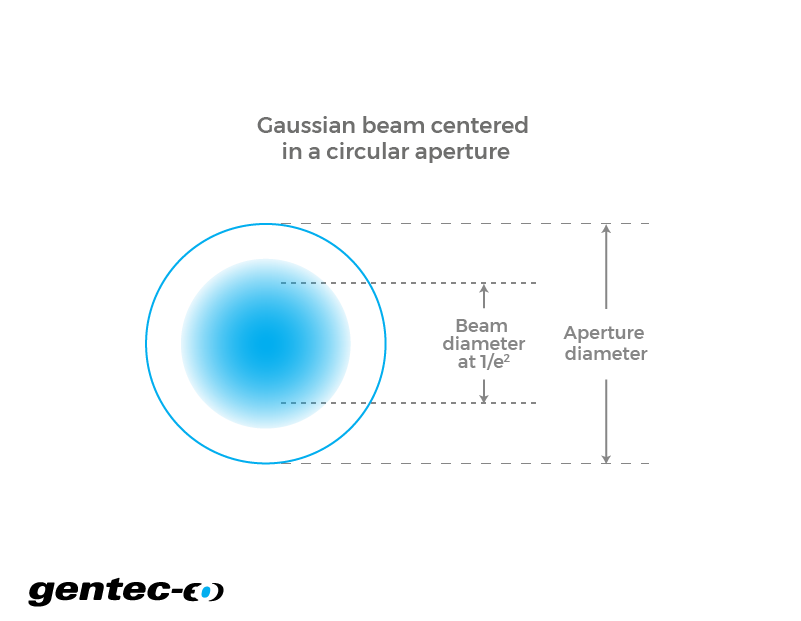EN

# Aperture transmission

Calculate a laser beam transmission through an aperture with this free online calculator.

-

- %

## How does the laser beam aperture calculator work?

When you consider a circular Gaussian laser beam, each wavefront that it creates has (theoretically) a certain value of energy everywhere in the universe. So, if the laser beam goes through an aperture, no matter how big the aperture actually is, there is a certain amount of energy from the incident laser beam that does not go through. Knowing the diameter at 1/e² of your laser beam will then make it possible to calculate the resulting power that is passing through an aperture. As one could expect, the bigger the aperture, the more negligible becomes the proportion of the power that is blocked. This is of importance when using detectors, mainly because having an inappropriate aperture dimension would lead to inaccurate measurements of either power or energy. This is also true when thinking about optical designs of new technologies. Finally, depending on how crucial your accuracy of measurement needs to be (and how good you are at centering your beam on the detector), as a rule of thumb, aim for an aperture that is double the size of the beam at 1/e². At this point, you’ll already have more than 99.9% of that incident beam power going through the aperture (if perfectly centered).

Click to
enlarge## Formulas

The formulas describe the behavior of a perfectly circular Gaussian laser beam. In such, they represent an approximation of the values one would obtain in real conditions. The diameter value that is used for the beam refers to the 1/e² parameter. We also assume that the laser beam is perfectly centered in the aperture, making the beam and the aperture two concentric circles. Also, one should know that the diameter of a Gaussian beam is a function of z. Therefore, its value is different according to the point where it is measured. This refers to more complex physicals concepts relative to the propagation of a Gaussian beam in space which will not be discussed here.

ref: Saleh, B. E. A., & Teich, M. C. (2007). Fundamentals of Photonics (2nd ed.). Wiley-Interscience.

$$\text{Transmitted power}(W) = \text{Beam initial power}(W) \times \left(1 - e^{\frac{-2 \times (\text{aperture radius})^2}{(\text{beam radius at aperture})^2}}\right)$$

Right-click
to copy

$$\text{% Transmission} = \frac{\text{Transmitted power}(W)}{\text{Beam initial power}(W)} \times 100$$

Right-click
to copy